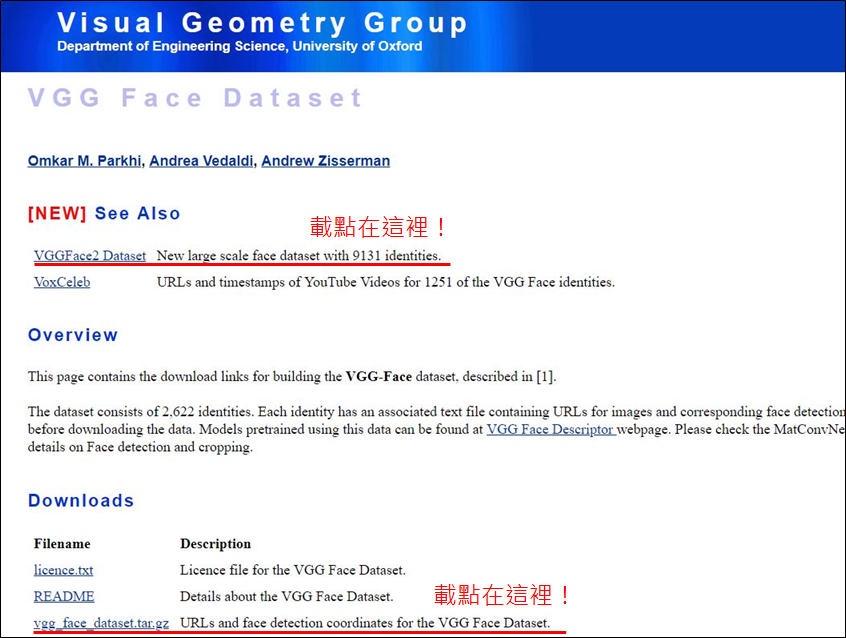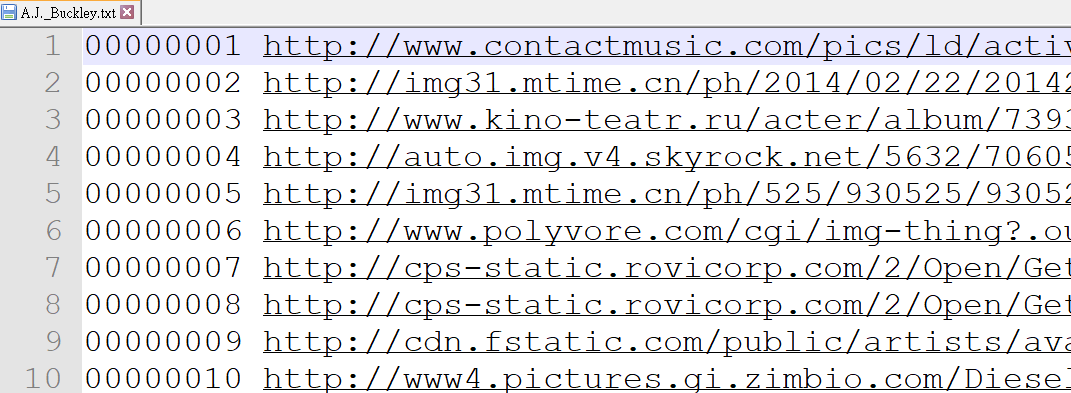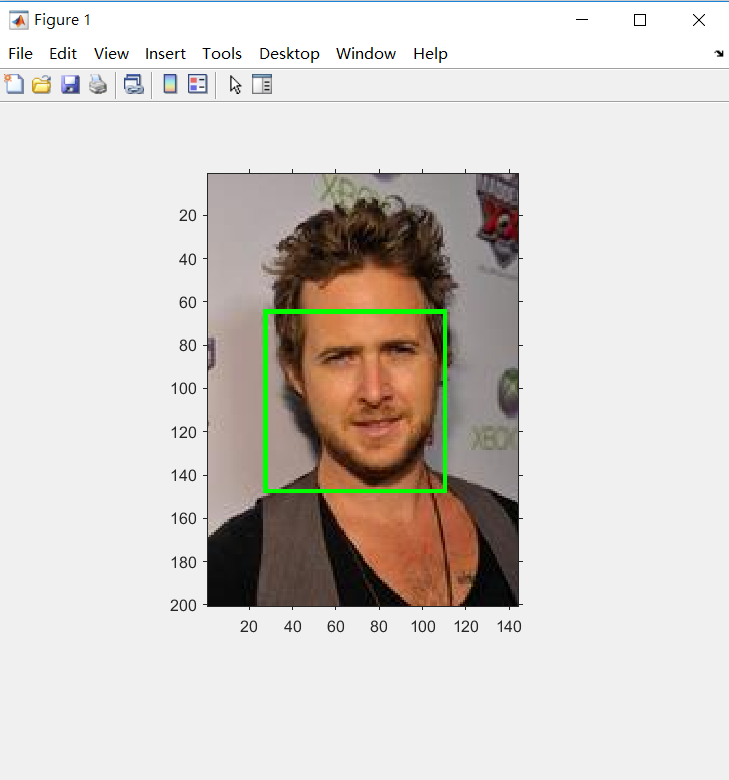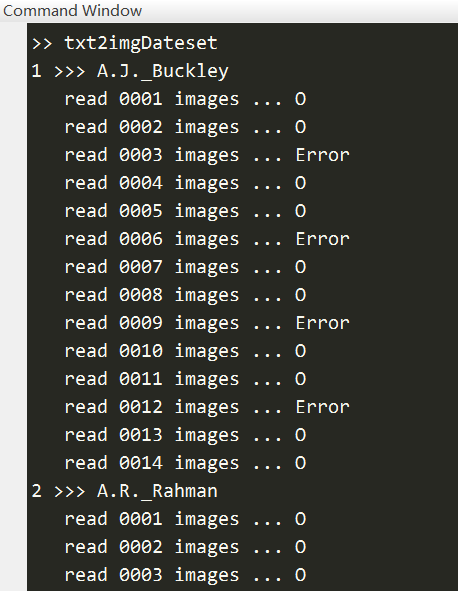### 【MATLAB】建立 VGG Face Dataset 人臉資料集

• 510
• 0
• 2019-05-24

VGG-Face dataset 是由牛津大學視覺幾何組提供的人臉資料集。## 1. 資料介紹

VGG-Face 解壓縮後，資料夾內可以看到三個檔案。00000001 ...(網址太長，略)
00000002 ...(略)
00000003 ...(略)
00000004 ...(略)
00000005 http://img31.mtime.cn/ph/525/930525/930525.jpg 28.19 65.33 111.43 148.57 4.00 3.60 0
...

1.編號。
2.圖片網址。
3.人臉方框(left, top, right, bottom)，共 4 個數字。
4.姿勢，其值大於2表示正面，小於2表示側面。
5.偵測角度。
6.是否存在於專業資料庫。

% MATLAB code

bbox = [28.19 65.33 111.43 148.57];
bbox = [bbox(1), bbox(2), bbox(3)-bbox(1), bbox(4)-bbox(2)];

I1 = insertShape(I, 'Rectangle', bbox, 'Color', 'g', 'LineWidth', 2);
imshow(I1)## 2. 批次處理建檔

function txt2imgDateset
% MATLAB code
%
% Shayne, 2019.05.15

%% 載入資料夾
VGG_Dataset = datastore('vgg_face_dataset\files', 'IncludeSubfolders', 1);

% 計算檔案數量
numFiles = length(VGG_Dataset.Files);

% 建立影像資料夾
imgDataset = [pwd, '\dataset\'];
if ~exist(imgDataset, 'dir')
mkdir(imgDataset)
end

%% 下載資料

% 設定讀取影像數量
max_file_number = 10;

% 紀錄影像資訊
image_status = struct([]);
idx = 1;

for i = 1:numFiles
% check folder
str = strsplit(VGG_Dataset.Files{i}, {'\'});
label = strrep(str{end}, '.txt', '');
if ~exist([imgDataset, label], 'dir')
mkdir([imgDataset, label])
end

fprintf('%d >>> %s\n', i, label)

data = table2cell(data);
numData = size(data, 1);

totalimgs = 1;
for j = 1:numData

if totalimgs > max_file_number
break
end

try
fprintf('   read %04d images ...', j)

img_path = [imgDataset, label, '\img', num2str(j), '.jpg'];
imwrite(I, img_path)

totalimgs = totalimgs + 1;

% save the information of image
image_status(idx).path = img_path;
image_status(idx).bbox = [data{j, 3}, data{j, 4}, data{j, 5}-data{j, 3}, data{j, 6}-data{j, 4}];
idx = idx + 1;

save image_status image_status
fprintf(' O\n')
catch
fprintf(' Error\n')
end
end
end